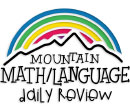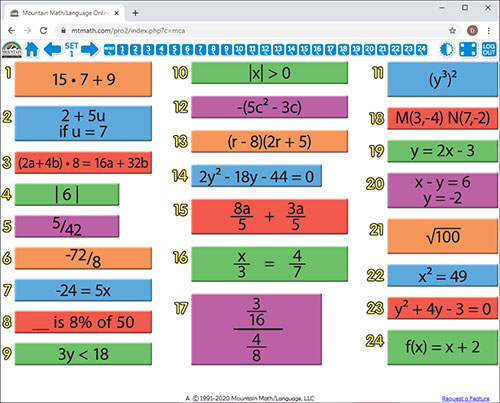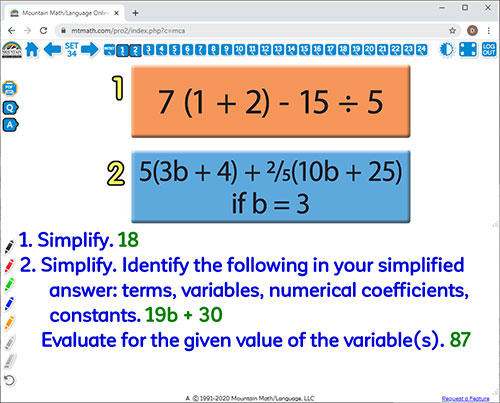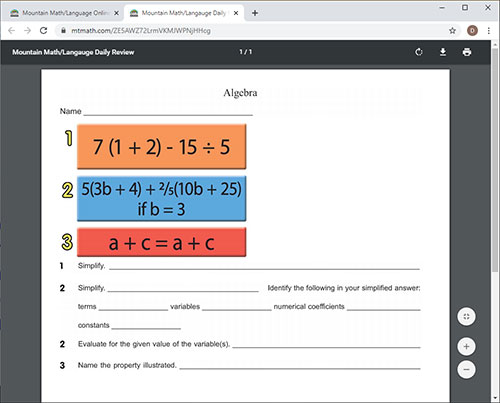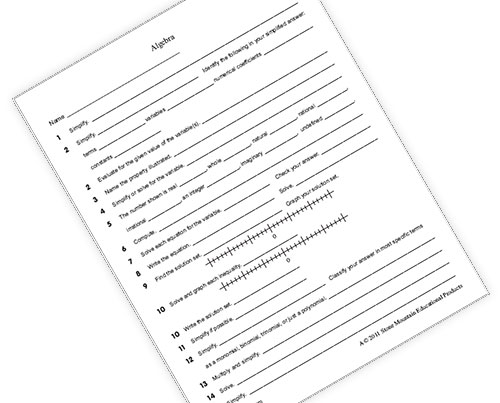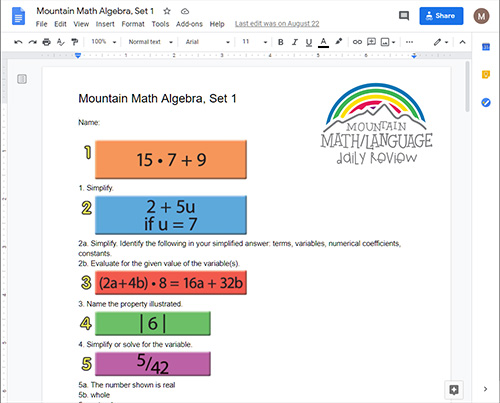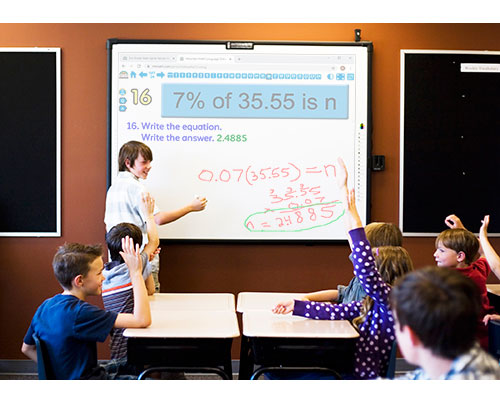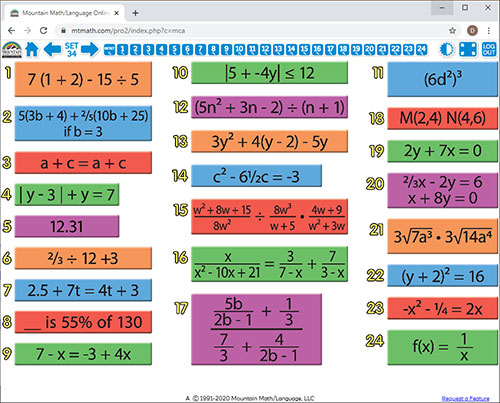WHAT YOU GET:
• 32 WEEKS OF DAILY REVIEW
• GOOGLE DOCS PROBLEM SETS
• GOOGLE FORMS PROBLEM SETS
• CUSTOMIZABLE PDF PROBLEM SETS
• STUDENT RESPONSE SHEET FILE AND ANSWER KEY
• 12 MONTH ACCESS FOR YOU AND YOUR CLASSROOM
(1 ONLINE SUBSCRIPTION = 1 TEACHER + 1 CLASSROOM)
• KNOWLEDGEABLE STAFF FOR ANY ASSISTANCE

This ALGEBRA MOUNTAIN MATH ONLINE SUBSCRIPTION is DAILY REVIEW your students need to MASTER the concepts you have taught.

Research has proven that LONG-TERM MASTERY comes from SPIRAL REVIEW, also called DISTRIBUTED PRACTICE and SPACED REPETITION.

The ONLINE SUBSCRIPTION is designed to be projected onto an interactive white board, creating a virtual board. It can be viewed by the entire classroom. Answers can be displayed onto the board at the click of a button. Up to seven questions can be isolated and magnified.

Grade level is configured on first log in.

Most devices with a modern web browser are supported (Windows, OSX, Linux, iOS, Android, Chrome OS).

30-Day Money-Back Guarantee.
1. Order of operations - including parentheses and exponents.
2. Evaluate algebraic expressions that include fractions, decimals, exponents, and integers.
Combining like terms including integers and parentheses.
Identify terms, variables, numerical coefficients, and constants.
3. Identify properties - Commutative, Associative, Distributive, Multiplicative and Additive Identity, Multiplicative and Additive Inverse, Symmetric, Transitive, Reflexive, and Substitution.
4. Absolute value.
Solve equations with Absolute Value.
5. Number concepts - Real, Whole, Natural, Rational, Irrational, Integer, Imaginary, Undefined.
6. Addition, subtraction, multiplication, and division of integers - including whole numbers, fractions, and decimals.
7. Solve one and two step equations with integers, fractions, and decimals.
Solve one and two step equations with parentheses.
Solve equations with variables on both sides.
8. Percent equations.
9. Solve and graph solution sets to equations and inequalities.
10. Conjunction and disjunction inequalities.
Solve absolute value inequalities.
11. Properties of exponents.
12. Addition, subtraction, multiplication, and division of polynomials.
Simplify polynomials.
Classify polynomials.
13. Multiply polynomials.
Use FOIL to multiply two binomials.
14. Factor polynomials completely including Greatest Common Factor.
Set quadratic and cubic equations up in standard form.
Solve quadratic and cubic equations by factoring.
15. Addition, subtraction, multiplication, and division of rational expressions.
Reduce rational expressions.
16. Solve proportions.
Solve rational expressions.
Solve literal equations.
17. Simplify complex rational expressions.
18. Graph ordered pairs on the coordinate (Cartesian) plane.
Identify the abscissa and ordinate in an ordered pair.
Identify the quadrant or axis an ordered pair lies in or on.
Determine the slope and the direction of a line.
19. Set equations of lines up in slope-intercept form.
Identify the slope and Y-intercept.
Graph lines using the slope intercept form - including vertical and horizontal lines.
Graph inequalities using slope intercept form.
20. Solve system of equations.
21. Simplify square roots.
Addition, subtraction, multiplication, and division of square roots.
Rationalize the denominator.
22. Solve square root equations.
Solve quadratic equations using square root properties.
23. Solve quadratic equations using the quadratic formula.
24. Functions.
ONLINE REVIEW VS.
CENTER EDITION VS.
BULLETIN BOARD KIT
ONLINE REVIEW
Is projected onto an interactive white board, creating a virtual board. It can be viewed by the entire classroom. Answers can be displayed onto the board at the click of a button. Up to seven questions can be isolated and magnified.

CENTER EDITION
Is organized on a 13"x19" flip chart. It can be viewed by 5-6 students at a time. It was designed for small group review, such as rotations, scoots, centers, or math-around-the-classroom. It has an answer key, heavy-duty stand, and high-gauged coil.

BULLETIN BOARD KIT
It is designed for total classroom view. It hangs on bulletin boards, walls, white boards, pocket charts, and science display boards. Once hung, it can remain displayed for the entire year. The cards can be interchanged, creating flexible and adjustable DAILY REVIEW.
ONLINE REVIEW PRICES
\$0.00
Free Trial

\$59.95
1 Year of Math Review

\$99.95
1 Year of Math and Language Review

\$99.95
1 Year of Review with 3 Grade Levels

\$59.95
1 Year Renewal
(separate multiple usernames with a comma)

Online Review   |   Math Algebra
To preview a grade level, click on the grade icon.

This will display covered concepts, images, and more.

## What teachers are saying about Mountain Math and Mountain Language:

"I used Mountain Math and Mountain Language in my second grade ESL classroom. For the first few months, I didn't use it because I had so many materials and resources. I thought that it would be too much work. After I went through the kits, I realized how good they were! Both the math and language cover many skills. You...(more)"
-Tiffany Weston, Houston, Texas, Third Grade Math/Science/Social Studies